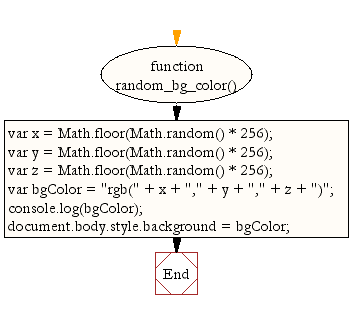# JavaScript: Create random background color

## JavaScript Math: Exercise-40 with Solution

Write a JavaScript function to create random background color.

Pictorial Presentation:Sample Solution:-

HTML Code:

``````<!DOCTYPE html>
<html>
<meta charset="utf-8">
<title>JavaScript function to create random background color</title>
<body>

</body>
</html>
```
```

JavaScript Code:

``````function random_bg_color() {
var x = Math.floor(Math.random() * 256);
var y = Math.floor(Math.random() * 256);
var z = Math.floor(Math.random() * 256);
var bgColor = "rgb(" + x + "," + y + "," + z + ")";
console.log(bgColor);

document.body.style.background = bgColor;
}

random_bg_color();
```
```

Sample Output:

```rgb(251,34,92)
```

Flowchart:Live Demo:

See the Pen javascript-math-exercise-40 by w3resource (@w3resource) on CodePen.

Improve this sample solution and post your code through Disqus

What is the difficulty level of this exercise?

﻿

## JavaScript: Tips of the Day

```const name = 'Minta Lofton';